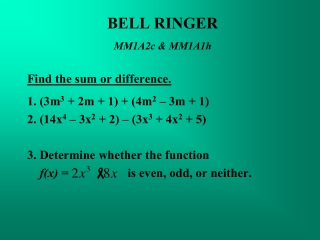DownloadDownload PresentationBELL RINGER MM1A2c & MM1A1h

# BELL RINGER MM1A2c & MM1A1h

Download Presentation## BELL RINGER MM1A2c & MM1A1h

- - - - - - - - - - - - - - - - - - - - - - - - - - - E N D - - - - - - - - - - - - - - - - - - - - - - - - - - -
##### Presentation Transcript

1. BELL RINGER MM1A2c & MM1A1h Find the sum or difference. 1. (3m3 + 2m + 1) + (4m2 – 3m + 1) 2. (14x4 – 3x2 + 2) – (3x3 + 4x2 + 5) 3. Determine whether the function f(x) = is even, odd, or neither.

2. Essential Question

3. Daily Standard & Essential Question • MM1A2c :Add, subtract, multiply, and divide polynomials • MM1A2g: use area and volume models for polynomials arithmetic • Essential Question: What are the three special products and how can you quickly find each one?

4. There are formulas (shortcuts) that work for certain polynomial multiplication problems. (a + b)2 = a2 + 2ab + b2(a - b)2 = a2 – 2ab + b2(a - b)(a + b) = a2 - b2 Being able to use these formulas will help you in the future when you have to factor. If you do not remember the formulas, you can always multiply using distributive, FOIL, or the area model method.

5. Let’s try one!1) Multiply: (x + 4)2 You can multiply this by rewriting this as (x + 4)(x + 4) OR You can use the following rule as a shortcut: (a + b)2 = a2 + 2ab + b2 For comparison, I’ll show you both ways.

6. First terms: Outer terms: Inner terms: Last terms: Combine like terms. x2 +8x + 16 1) Multiply (x + 4)(x + 4) Notice you have two of the same answer? x2 +4x +4x x2 +4x +16 +4x +16 Now let’s do it with the shortcut!

7. That’s why the 2 is in the formula! 1) Multiply: (x + 4)2 using (a + b)2 = a2 + 2ab + b2 a is the first term, b is the second term (x + 4)2 a = x and b = 4 Plug into the formulaa2 + 2ab + b2 (x)2 + 2(x)(4) + (4)2 Simplify. x2 + 8x+ 16 This is the same answer!

8. 2) Multiply: (3x + 2y)2using (a + b)2 = a2 + 2ab + b2 (3x + 2y)2 a = 3x and b = 2y Plug into the formulaa2 + 2ab + b2 (3x)2 + 2(3x)(2y) + (2y)2 Simplify 9x2 + 12xy +4y2

9. Multiply: (x – 5)2 using (a – b)2 = a2–2ab + b2Everything is the same except the signs! (x)2 – 2(x)(5) + (5)2 x2 – 10x + 25 4) Multiply: (4x – y)2 (4x)2 – 2(4x)(y) + (y)2 16x2 – 8xy + y2

10. First terms: Outer terms: Inner terms: Last terms: Combine like terms. x2 – 9 5) Multiply (x – 3)(x + 3) Notice the middle terms eliminate each other! x2 +3x -3x x2 -3x -9 +3x -9 This is called the difference of squares.

11. 5) Multiply (x – 3)(x + 3) using (a – b)(a + b) = a2 – b2 You can only use this rule when the binomials are exactly the same except for the sign. (x – 3)(x + 3) a = x and b = 3 (x)2 – (3)2 x2 – 9

12. 6) Multiply: (y – 2)(y + 2) (y)2 – (2)2 y2 – 4 7) Multiply: (5a + 6b)(5a – 6b) (5a)2 – (6b)2 25a2 – 36b2

13. HomeworkTextbook Page 70; 2 – 20 Even